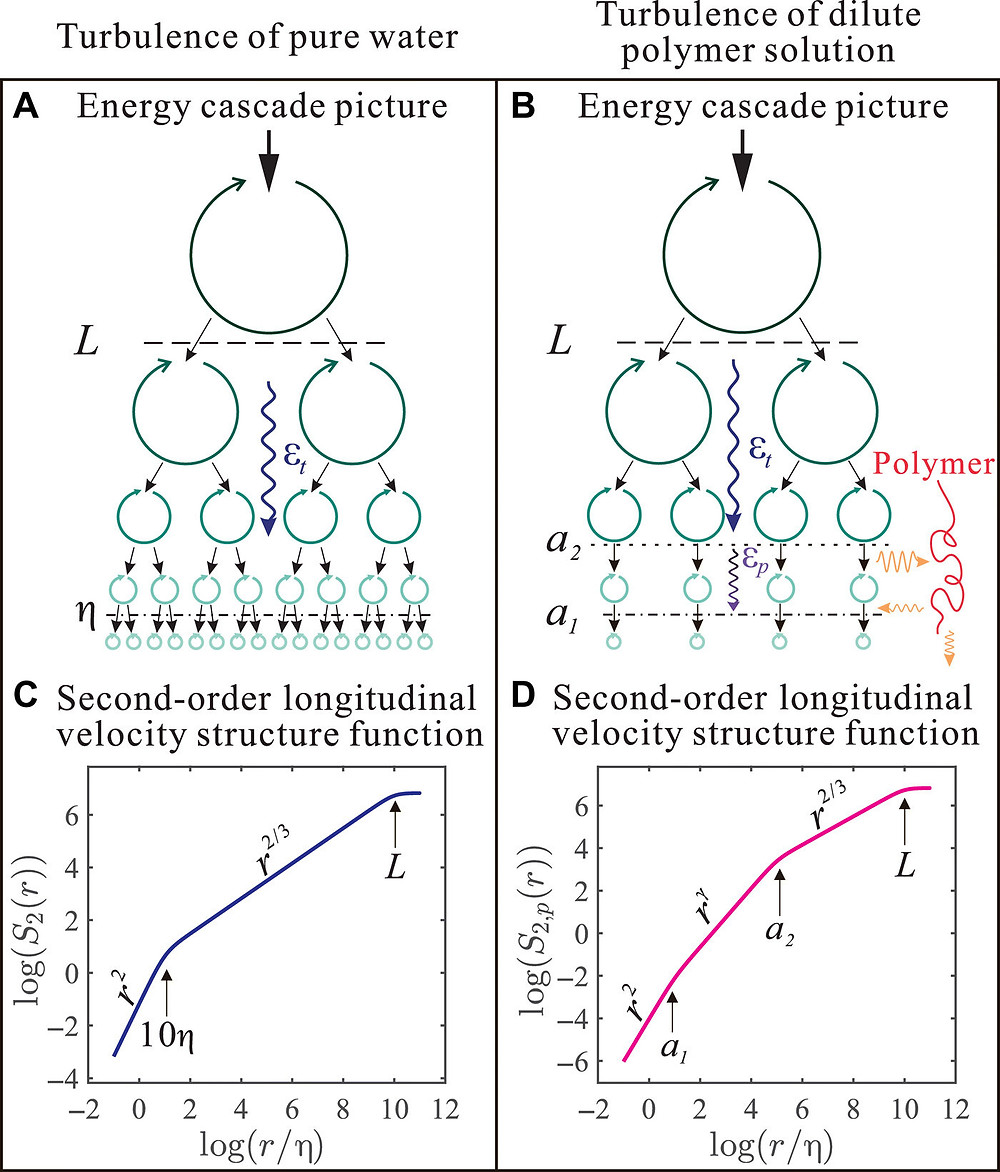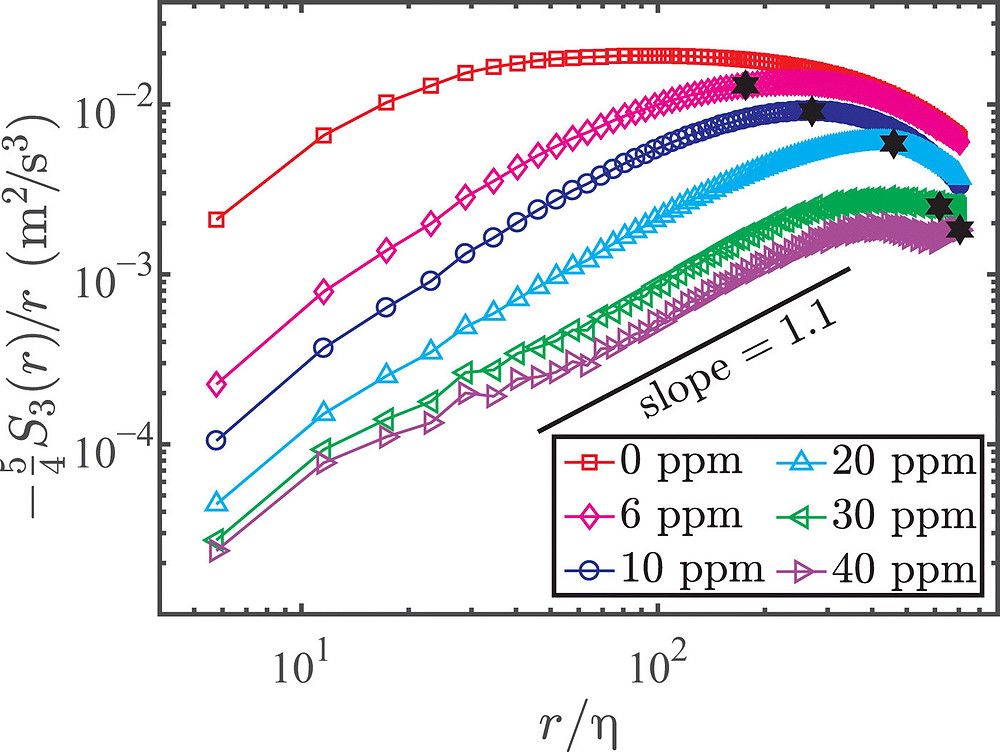Search

# Experimental observation of the elastic range scaling in turbulent flow with polymer additivesPhysical picture of the turbulent energy cascade and its manifestation on the second-order longitudinal velocity structure function (VSF). (A and B) Cartoons showing the physical picture of the energy cascade in turbulent flow of pure water and dilute polymer solution. (C and D) The second-order longitudinal VSF in turbulent flow of pure water case and dilute polymer solution case. Credit: Science Advances, doi: 10.1126/sciadv.abd3525

When long-chain flexible polymers are dissolved in a turbulent flow, the flow properties can be changed drastically by reducing the drag and enhancing the mixing. A fundamental riddle in materials science is to understand how these polymer additives interact with different spatial scales in turbulent flow to alter the turbulence energy transfer. In a new report now on Science Advances, Yi-Bao Zhang and a research team showed how turbulent kinetic energy could be transferred across different scales in the presence of polymer additives. The team noted the emergence of a previously unidentified scaling range known as the elastic range, where an increased amount of energy could be transferred by the elasticity of the polymers. The findings have important applications across many turbulent systems, including turbulence in plasmas or superfluids.

Flow properties and velocity structure-function (VSF)

Materials scientists have shown how dissolving a tiny amount of long-chain flexible polymer in a fluid could change the flow properties. Reynolds number helps predict flow patterns under different fluid flow situations. At low Reynolds, normal fluid flow is stable and laminar, and the addition of polymers can induce strong fluctuations to create elastic turbulence. High-Reynolds number turbulent flows can result in substantial reduction of drag and the enhancement or reduction of convective heat transfer. Researchers aim to understand the interaction between polymers and the turbulence cascade for theoretical reasons and practical applications. It is presently critical to comprehensively measure the energy spectra or the velocity structure-function (VSF) in turbulent flows with polymer additives. In this report, Zhang et al. detailed experimental observation of the new elastic range in a laboratory turbulent flow setup and measured the scaling of the velocity structure-function in the new elastic range, which diverged from any existing theory.Second-order longitudinal VSFs [S2(r)] for pure water and dilute polymer solutions at Rλ=530. (A) S2(r) and r are normalized by u2η and η, respectively. Here, Rλ, η, and uη are from the pure water case. The solid curves are fits to the parameterization function (Eq. 2). For the sake of clarity, lower ϕ data have been shifted upward by 100.15 with respect to its higher ϕ neighbor. (B) The same data as in (A) but S2,p(r) is compensated by elastic range scaling r1.38. For the sake of clarity, each dataset has been shifted up by 0.25 with respect to its higher ϕ neighbor. The cyan and magenta pentacles show the crossover scales a1 between the dissipation and the elastic ranges and a2 between the elastic and the inertial ranges, respectively. (C) The same data as in (A) but S2, p(r) is compensated by its exact form in the elastic range given by the parameterization: s2xxa0.621r1.38, and r is normalized by a2. The solid curve is (r/a2)−0.71. Credit: Science Advances, doi: 10.1126/sciadv.abd3525

The experimental setup

The scientists generated the turbulent flow in a von Kármán swirling apparatus containing two counter-rotating disks enclosed in a cylindrical tank filled with 100 L of water or polymer solutions. They measured the three components of fluid velocity in a central planar passing through the axis of the tank using a stereoscopic particle image velocimetry (PIV) system. According to the measurements, the flow near the center of the tank was nearly homogeneous and isotropic for both flows with water and with dilute solutions of long-chain polymers in water. The scientists used polyacrylamide (PAM) for the polymers during the experiments. The team noted a Reynolds number for the pure water to range from 340 to 350, indicating a fully developed inertial range in turbulence. At equilibrium, the polymers remained in the coiled state. During weak flow in the solution, the polymer remained in the coiled state with negligible effect on the flow. Comparatively, during intense flow, the polymers stretched to store elastic energy for release into the fluid. The fluid then displayed viscoelastic behavior. During turbulent flows, they characterized the transition using the Weissenberg number to measure the polymer relaxation time relative to the turbulence time scale. For the polymers to be stretched by the flow, the Weissenberg number had to be larger than unity. During the measurements, Zhang et al. only considered the interaction between the fluid and single polymer, while neglecting direct polymer-polymer interactions.The local turbulence kinetic energy transfer rate determined from the third-order longitudinal VSF. Compensated third-order longitudinal VSF −54S3(r)/r=ε(r) as a function of r/η for pure water case and the polymer solution cases at Rλ = 480. The black pentacles show the crossover scale a2 between the elastic and the inertial ranges. Credit: Science Advances, doi: 10.1126/sciadv.abd3525

Measuring the elastic range

To then quantify the boundary of the elastic range, the researchers adapted an analytical form of the second-order longitudinal velocity structure-function (VSF) for Newtonian turbulence proposed by Batchelor et al. As the polymer concentration in the sample increased, the measured mean square of the derivative of the longitudinal velocity decreased, indicating that the energy dissipated by viscosity at very small scales—consistent with previous experiments and numerical simulations. The decreased viscous dissipation with polymer concentration alongside the independence of the turbulence energy transfer rate at larger scales indicated the energy transfer rate in the elastic range to vary non-trivially. The team therefore next investigated methods to obtain the energy transfer rate with a setup that drew incrementally more energy into the elastic energy of the polymer due to interactions between turbulent eddies and polymer elasticity.

The crossover scales

Zhang et al. then identified the elastic range and examined the crossover scale between the elastic range and the dissipation range (termed a1), followed by the crossover scale between the elastic range and inertial range (termed a2). They then studied how the two crossover scales varied with control parameters. The crossover scale between the elastic range and the dissipation range appeared to decrease slightly with polymer concentration; however, the team credited this to likely contamination due to poor spatial resolution of the particle image velocimetry measurements. The scientists then corrected the observed inaccuracy as a function of polymer-concentration and showed that for small polymer concentrations, the crossover scale between the elastic range and inertial range was very small.The variation of the crossover scales a1 between the dissipation and the elastic ranges and a2 between the elastic and the inertial ranges. a1 and a2 as functions of ϕ for four different Rλ. Here, a1 and a2 are normalized by η from the pure water case. a1 at lower concentration from previous experiments [Rλ = 270, 340, 360 data from and Rλ = 350 data from] are also plotted for comparison. The slope = 0.8 straight line is to show that overall a2 scales with ϕ0.8, while the slope = 0.4 straight line is to compare the data in the low concentration range with the prediction rε ∼ ϕ0.4.

Scaling of the high-order velocity structure-function

The team also investigated the problem of turbulence flow to scale the high-order velocity structure-function (VSF) on the inertial range with water and polymer additives. The resulting similarities in behavior showed how the elastic range of the energy transfer through scales was altered by polymers. The team expects to observe common features between Newtonian turbulence and polymeric turbulence. The results showed excellent agreement between the data and prediction to show how the energy transfer was substantially altered by polymers in the elastic range. Meanwhile, the fluctuating local energy transfer followed similar statistical descriptions as that of Newtonian turbulence.Scaling of high-order VSF in the elastic range at Rλ=480 and ϕ=40 ppm. (A) The nth-order (n=1 to 8, from top to bottom) longitudinal VSF in the polymer solution Sn, p(r) as a function of r/a2 (or r/a1, upper axis), the range between the two vertical dashed lines is the elastic range, the scaling exponent ξp(n) is obtained from the power-law fitting to this range. The absolute values of the velocity increments are used to calculate the VSF. (B) Local slope d[ log (Sn, p(r))]/d[ log (r)] of Sn, p(r) for n= 1 to 8 (from bottom to top) as a function of r/a2 (or r/a1, upper axis). The two vertical dashed lines mark the region where the local slope is nearly constant. The horizontal solid lines represent the average value within the two dashed lines. (C) Elastic range scaling exponents ξp as a function of n. ξp obtained from both the direct fitting and the local slope are plotted. The inertial range scaling exponents for pure water ξw(n) is also plotted for comparison. The dashed line is ξp(n) = 0.7n. The solid line is the K41 prediction, i.e., ξw(n) = n/3. (D) Δξ(n) = ξp(n) − ξw(n) as a function of n. The solid line is Δξ(n) = 1.1n/3.

Outlook

In this way, Yi-Bao Zhang and colleagues experimentally observed the scaling of the elastic range in the turbulent flow with polymer additives. They measured the turbulent kinetic energy transfer in the presence of polymer additives. As the energy flux through the turbulent flow decreased, the energy flux through the elastic degree of freedom of polymers increased. The study shed new light to conduct further theoretical and numerical investigations on the interaction between the elasticity of polymer additives and turbulent eddies. These experimental processes can be noted in practice within physical mechanisms such as electromagnetic interactions in plasmas and Alfvén waves in superfluids.

## Recent Posts

See All

#### Sloshing electrons in a charge density wave

In the latest edition of Physical Review B, UvA Ph.D. candidate Xuanbo Feng (QuSoft and IoP) and colleagues write about their recent experim